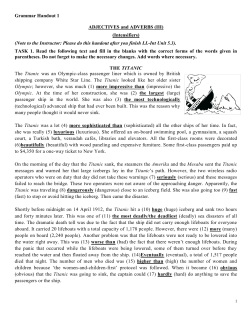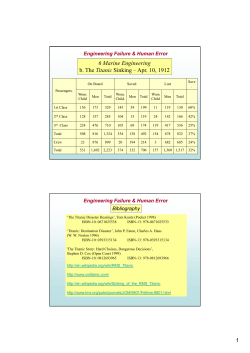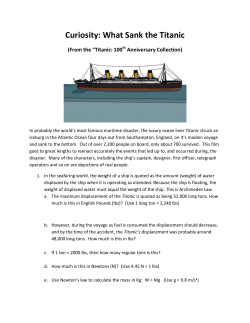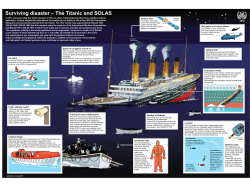# R Introduction Workshop Slides

```Introduction to R
http://dataservices.gmu.edu/workshop/r
1. Create a folder with your name on the S: Drive
2. Copy titanic.csv from R Workshop Files to that folder
History
R ≈ S ≈ S-Plus
Open Source, Free
http://www.r-project.org/
CRAN = Comprehensive R Archive Network
RStudio: www.rstudio.com
R Console
Console
>
+
prompt for new command
waiting for rest of command
Console
↑[Up] to get previous command
History
Double-click to put in console
Type in everything in Courier New (only):
3+2
32
Script Window
or
File | New … | R Script
Objects
nine <- 9
nine
three <three
nine / 3
my.school <- "gmu"
my.school
Historical Conventions
Use <- to assign values
Use . to separate names
Current Capabilities
= is okay now in most cases
_ is okay now in most cases
RStudio:
Press Alt - (minus) to insert "assignment operator"
Press Ctrl-Enter to run the current line
Environment
History
Double-click
to put in Console
Other Stuff
Files
Plots
Packages
Help
Packages
Packages must be both Installed and Loaded
To Install:
install.packages("name")
Install
library( name )
or, require( name )
or, check the box
Confirm these are installed:
dplyr
tidyr
descr
ggplot2
Installed
Functions
Function
Positional
Argument
Named
Argument
Named
Argument
becomes the convenience function…
Get help for any function with ? ?read.csv
(to use \ , type \\ )
Help
or
Object Types
Vectors & Lists
numbers <- c(101,102,103,104,105)
numbers <- 101:105
the same
numbers <- c(101:104,105)
numbers[ 2 ]
numbers[ c(2,4,5)]
numbers[-c(2,4,5)]
numbers[ numbers > 102 ]
Vector  Variable
Data Frames
int / num = Numeric
(Interval / Ratio)
str(titanic)
think structure
Factor = Categorical
(Nominal /Ordinal )
titanic\$pclass
as.is = "name")
Object Types
Numbers, Strings
Vectors, Lists
Data
– data.frame
– data.table
package
dplyr
 tbl_df
package
History Fact
who created dplyr,
works at RStudio
 tbl_dt
titanic
library(dplyr)
titanic <- tbl_df(titanic)
titanic
str(titanic)
Factors - Categorical Variables
titanic\$pclass <- factor(
titanic\$pclass,
levels = c(1,2,3),
labels = c("1st Class",
"2nd Class",
"3rd Class"),
ordered = TRUE
)
current values
labels in the
same order
ordinal variable
labels(titanic\$embarked) < c("",
"Cherbourg","Queenstown","Southampton")
NA and NULL
Delete Variable
titanic\$sibsp
<-
NULL
Set Values to Missing
titanic\$age[titanic\$age == 99] <- NA
same thing while reading in data:
titanic <- read.csv("S:/name/titanic.csv", na.strings = "99")
Ignore NAs Option
na.rm = TRUE
Review
Words with Stuff
word
(Object)
word[ stuff ] (Object Part)
word( stuff ) (Function)
"word"
Words that are not Objects
TRUE or T
FALSE or F
NaN (Not a Number)
NA (Not Available)
(String)
NULL (Empty)
Inf
(Infinity)
Descriptive Statistics
summary(titanic)
descr Package
library(descr)
freq(titanic\$pclass)
freq(titanic\$age)
CrossTable(titanic\$pclass, titanic\$survived)
CrossTable(titanic\$pclass, titanic\$survived,
prop.t = F,
prop.c = F,
prop.r = T,
T is default for all
digits = 2
)
ggplot2
library(ggplot2)
qplot(pclass, fill=survived, data=titanic)
titanic\$survived <factor(titanic\$survived,
labels = c("Died","Survived")
)
full documentation: http://ggplot2.org/
alternative: lattice
More with qplot
qplot(age, data=titanic)
qplot(age, data=titanic ,
fill = survived,
alpha = I(0.3),
position = "identity")
qplot(age,fare,data=titanic)
qplot(age,fare,color=survived,data=titanic)
R Markdown
Writing with R - Knitr
– html, pdf, docx, slides
– Descriptions with Code
– Descriptions with Output
Interactive Graphs
– Shiny
– ggvis
dplyr for data carpentry
select
filter
: Choose variables
: Choose cases
mutate
: Change values
summarize : Aggregate values
group_by : Create groups
arrange
: Order cases
History Fact
update of plyr for
data tables
Choose Variables
base
titanic\$name
titanic[,"name"]
titanic[,-"name"]
titanic[,c("age","gender")]
dplyr
select(
select(
select(
select(
select(
contains
starts_with
ends_with
matches
distinct
titanic,
titanic,
titanic,
titanic,
titanic,
name)
-name)
age, gender)
gender : pclass)
starts_with("p"))
Choose Cases
base
titanic[titanic\$age < 5 , ]
attach(titanic)
titanic[age < 5 , ]
titanic[age < 5 & is.na(age) == F , ]
titanic[(age<5|pclass==1)& is.na(age)==F , ]
dplyr
filter(titanic, age < 5 )
filter(titanic, age < 5, pclass == 1 )
filter(titanic, age < 5 | pclass == 1 )
Change data
base
titanic\$child <- titanic\$age <= 12
titanic\$totfam <- t\$sibsp + t\$parch
titanic\$bigfam <- titanic\$totfam > 4
dplyr
titanic <- mutate(titanic, child = age<=12)
titanic <- mutate(titanic,
totfam = sibsp + parch,
bigfam = totfam > 4
)
Chaining / Piping
%>%
RStudio: Ctrl+Shift+M
select(titanic, name, age)
vs
titanic %>% select(name, age)
titanic %>%
filter(age<5) %>%
select(name, age)
works anytime the 1st argument is the dataset
History Fact
from magrittr
originally %.%
Summarize
base
mean(titanic\$age )
mean(titanic\$age , na.rm = T )
sd(titanic\$age , na.rm = T )
dplyr
summarize(titanic, xbar=mean(age, na.rm=T))
summarize(titanic, n=n(), sd=sd(sibsp))
Other functions
dplyr
group_by
arrange
tidyr
gather
separate
bind_rows
Other packages from
lubridate
stringr
Pivot Table
library(dplyr)
library(tidyr)
titanic %>%
group_by(pclass, gender) %>%
summarize( pct=mean(survived) ) %>%
Statistical Analysis
http://www.ats.ucla.edu/stat/r/whatstat/whatstat.htm
Writing Models
y~x
y ~ x1 + x2 + x1 : x2
y ~ x1 * x2
~n|c
~
+
:
*
|
Simple Regression
2 variables + Interaction
2 variables + Interaction
n by each group of c
Separates Y from X (e.g., "predicted from")
adds another IV plus the interaction
creates subsets
t.test( fare ~ gender, data = titanic )
Analysis Objects
tt.aov <- aov( fare ~ gender*pclass,
data = titanic )
summary(tt.aov)
tt.glm <- glm( survived ~
pclass + gender + age + child
+ gender*pclass,
family = binomial,
data = titanic )
summary(tt.glm)
More with Analysis Objects
plot(tt.glm)
tt.pred <- predict(tt.glm)
tt.resid <- residuals(tt.glm)
plot(tt.pred, tt.resid)
compare to
qplot(tt.pred, tt.resid)
What now?
Analysis Environments
R Commander
Separate Interface
More/Better Statistics
www.rcommander.com
install.packages("Rcmdr")
library(Rcmdr)
Deducer
Adds to R Interface (not RStudio!)
Easier Data Management
www.deducer.org
install.packages("Deducer")
library(Deducer)
Data Mining GUI
install.packages("rattle")
require ("rattle")
rattle()
Tutorials
install.packages("swirl")
require ("swirl")
install_from_swirl("Course")
swirl()
http://swirlstats.com
Tutorials
http://dataservices.gmu.edu/software/r
http://tryr.codeschool.com/
https://www.datacamp.com/
Coursera, EdX, HarvardX
```